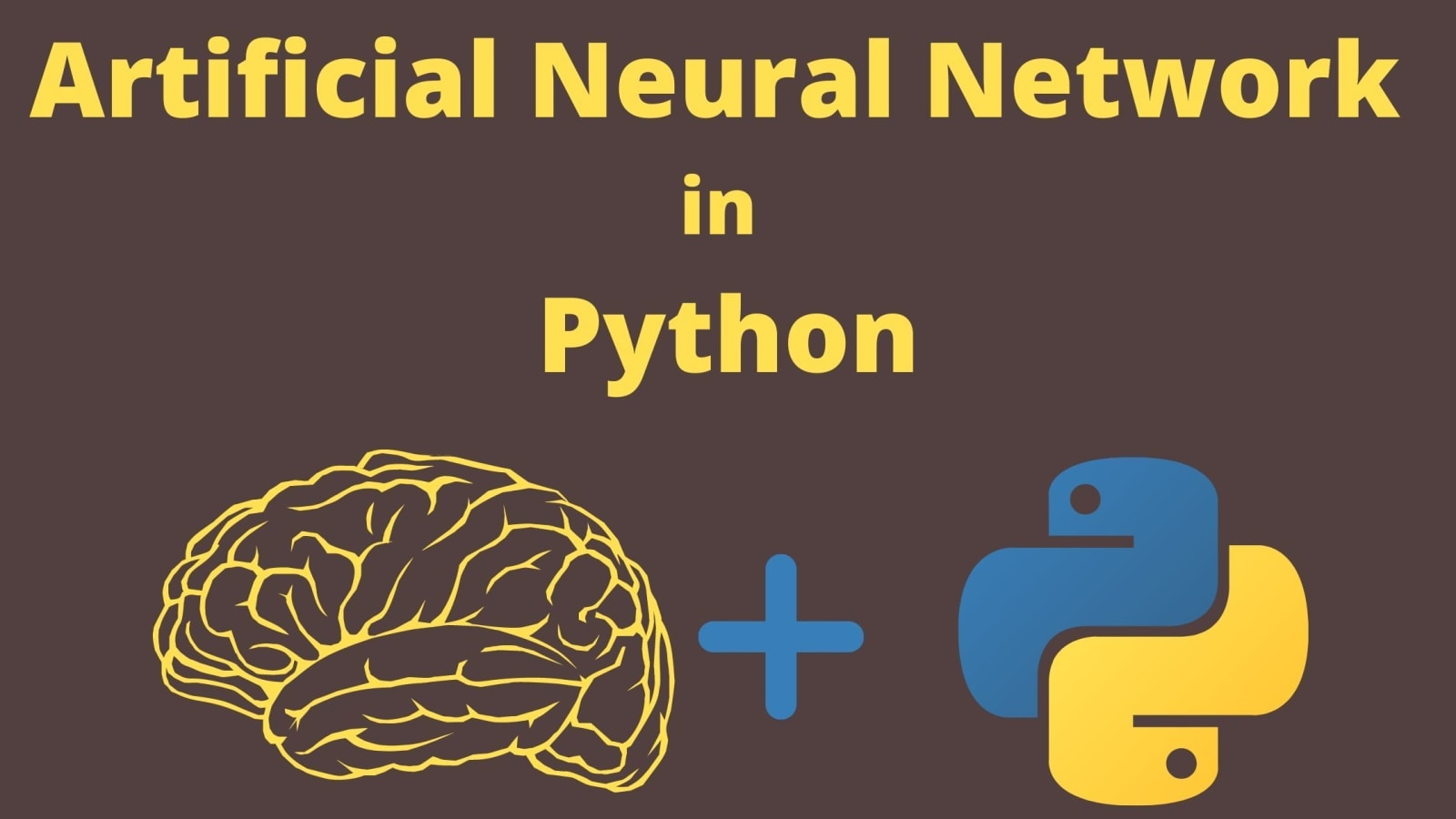# Create Simple neural Network with Python AI ProgrammingRead Time:3 Minute, 13 Second

Neural networks (NN), also called Artificial Neural Networks (ANN) are a subset of learning algorithms within the Machine Learning (ML) discipline, which are related to the concept of biological neural networks.

Most introductory texts to Neural Networks give brain analogies while describing them. Without delving into brain analogies. Andrey Bulezyuk-a German-based ML specialist with more than five years of experience, says that “neural networks are revolutionizing machine learning because they are capable of efficiently modeling sophisticated abstractions across an extensive range of disciplines and industries.”

Basically, the Artificial Neural Networks consist of the following components:

• An input layer that receives data and pass it on, x
• An arbitrary amount of hidden layers
• An output layer, ŷ
• A set of weights and biases between the layers, W and b
• A choice of deliberate activation function for every hidden layer, σ. In this simple neural network Python AI programming, let’s employ a Sigmoid activation function

There are various kinds of neural networks. Let’s create the feed-forward or perception neural networks. This type of ANN relays data directly from the front to the back.

Training the feed-forward neurons usually require back-propagation that offers the network with corresponding set of inputs and outputs. When the input data is transmitted into the neuron, it is processed, and an output is generated.

Below is a diagram, which shows the structure of a simple neural network:

And, the best way to know the functionalities of the neural networks takes place, is to learn how to develop one from scratch without taking help from any library.

# Creating a Neural Network Class

Now, let’s create a Neural Network class in Python to train the neuron to give an accurate prediction. The class will also have other helper functions.

Even though the usage is not done for the neural network library for this simple neural network example, the import of numpy library to assist with the calculations is necessary. The library gives the following four crucial methods:

1. exp—for generating the natural exponential
2. array—for generating a matrix
3. dot—for multiplying matrices
4. random—for generating random numbers.

Seed the random numbers to ensure their efficient distribution.

The output ŷ of a simple 2-layer Neural Network is:

One can notice that in the equation that is mentioned above, the weights W and the biases b are the only variables which affect the output ŷ. Naturally, the right values for the weights and biases determines the strength of the predictions. The process of fine-tuning the weights and biases from the input data is known as training the Neural Network. Every iteration of the training process consists of the following steps:

• Calculating the predicted output ŷ, known as feed forward
• Updating the weights and biases, known as back propagation

Building a Neural Network from scratch without a deep learning library such as TensorFlow. It is important to understand the inner working of a Neural Network is essential.

# Applying the Sigmoid function

The Sigmoid function draws a characteristic “S”-shaped curve, as an activation function to the neural network.

This function can map any value to a value from 0 to 1. It will be helpful to normalize the weighted sum of the inputs. The derivative of the Sigmoid function to help in computing the needed adjustments to the weights. The output of a Sigmoid function can be employed to generate its derivative. For instance, if the output variable is “x”, then its derivative will be x * (1-x).

The deep learning libraries such as TensorFlow and Keras make it simpler to develop deep nets without fully understanding the inner workings of a Neural Network.

# Wrapping up

With this knowledge, one can be ready to dive deeper into the world of Python AI programming. The process of training a neural network has application of operations to vectors.## About Post AuthorNext post Best Headshots Photographer Near Me: What You Should Know Before Hiring One

5 Star
0%
4 Star
0%
3 Star
0%
2 Star
0%
1 Star
0%